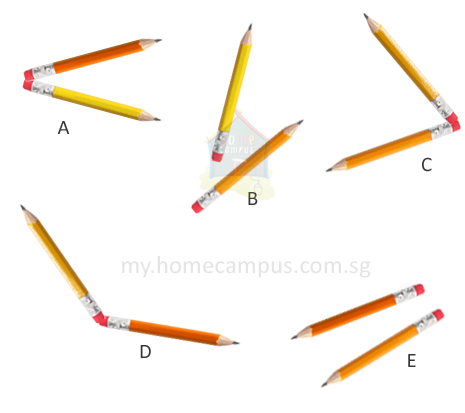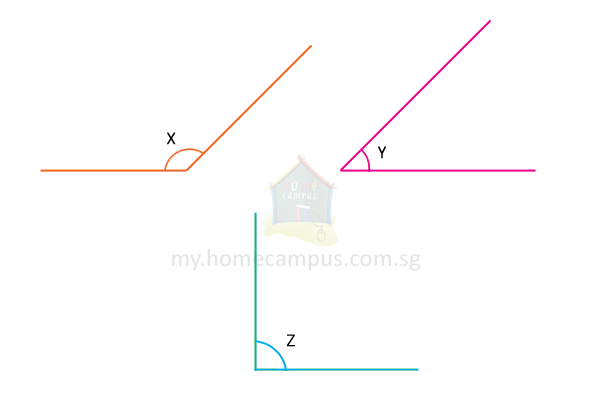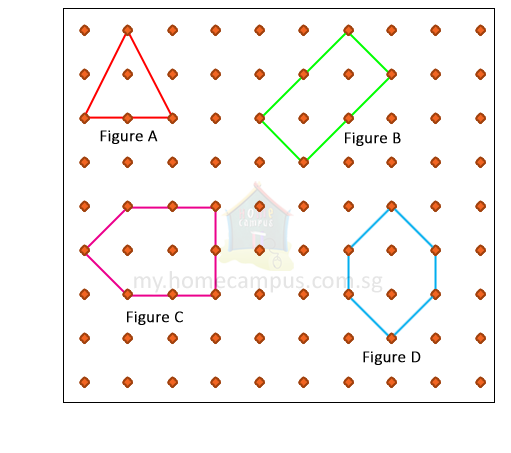## Identifying Angles in a Figure

Practice Unlimited Questions

#### 1. What is an angle?

An angle is the amount of turning between two lines meeting at a point.

#### 2. How are angles formed?

When 2 lines meet at a point, they form an angle at that point.

#### 3. How do we denote angles?

We denote angle using the symbol ∠.

#### 1. Ali bends a straw as below. Mark the angle formed by it.The bent straw (presented by the blue lines) forms an angle as marked by the red turning.

#### 2. Following are examples of angles on objects we see everyday.On each object, the angles are marked by the yellow turning.

#### 3. Here are some pairs of pencils placed in pairs. Which of these pairs form angles?Pairs A, C and D form angles.

#### c. Arrange the angles in order, beginning with the smallest.a. The lines forming Angle Y have the smallest turning.
So, ∠Y is the smallest angle.
b. The lines forming Angle X have the greatest turning.
So, ∠X is the greatest angle.
c. Beginning with the smallest, the angles can be arranged as below:
∠Y,     ∠Z,     ∠X

#### 5. Rayan makes some shapes as shown below. How many sides and angles does each shape have?Shape A has 3 sides and 3 angles.
Shape B has 4 sides and 4 angles.
Shape C has 5 sides and 5 angles.
Shape D has 6 sides and 6 angles.